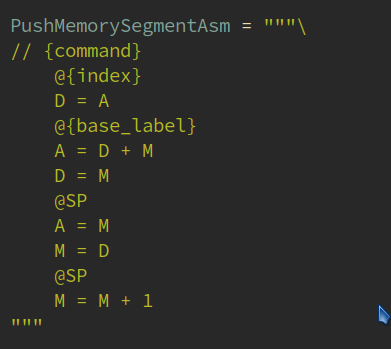# Emacs 要如何实现 Vscdoe 中 Python 那种折叠效果

3 个赞treesit对于python三种引号识别是很有问题的。

ts-fold 有個 treesit.el 的 fork, 看這裡. 看了一下代碼, 我以為大同小異.1 个赞

`ts-docstr-find-children` 改成 `ts-fold-find-children` 倒是可以实现大佬展示的效果，可以折叠三引号的文档字符串。但是对于下列的赋值语句，却没有折叠效果。

``````PopTempSegmentAsm = """\
// {command}
@{index}  // address = 5 + index
D = A
@5
D = D + A
@R13
M = D
@SP  // Get data in the stack
M = M - 1
A = M
D = M
@R13
A = M
M = D
"""
``````

``````expression_statement:
assignment:
identifier:
string:
``````

``````expression_statement:
string:
``````

1 个赞

``````(defun ts-fold-parsers-python ()
"Rule set for Python."
'((function_definition  . ts-fold-range-python-def)
(class_definition     . ts-fold-range-python-def)
(list                 . ts-fold-range-seq)
(dictionary           . ts-fold-range-seq)
(if_statement         . ts-fold-range-python-def)
(expression_statement . ts-fold-range-python-expression-statement)
(comment
. (lambda (node offset)
(ts-fold-range-line-comment node offset "#")))))
``````

``````(setq ts-fold-range-alist `((python-mode . ,(ts-fold-parsers-python))))  ; 更新一次
``````

Edit: `if`, `elif`, `else` 應該會更複雜一些. 你可以參考看看其他的語言怎麼做, Lua, C-macros, 等等. 或也可以等我抽空來弄!1 个赞

1 个赞